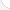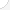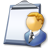Mechanical engineersDescription: Perform engineering duties in planning and designing tools, engines, machines, and other mechanically functioning equipment. Oversee installation, operation, maintenance, and repair of such equipment as centralized heat, gas, water, and steam systems.Source: Bureau of Labor Statistics, U.S. Department of Labor, Occupational Outlook Handbook, 2008-09 Edition at http://www.bls.gov/OCO/Complete Job Profile: http://www.bls.gov/oco/ocos027.htmSalary: \$50,001 or more per yearComments:

There are 56 math topics Mechanical engineers need to know.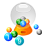Basic Math / Algebra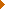FractionsDecimalsRatio and ProportionPercentCustomary MeasurementMetric MeasurementMeasurement ConversionBasic ProbabilityBasic StatisticsStatistical GraphingPowers and RootsNegative NumbersBasic Problem Solving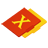First-Year AlgebraUsing FormulasLinear EquationsOperations with PolynomialsFactoring PolynomialsRational ExpressionsCoordinate Graphing 2DLinear SystemsAlgebraic Representation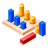GeometryBasic TerminologyAngle MeasurementCongruent TrianglesTriangle InequalitiesParallel LinesSimilarityPythagorean TheoremRight Triangle TrigonometryCirclesAreaVolumeTransformationsMake/Use 3D Drawings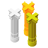Second-Year Algebra / TrigonometryFunctionsVariationLogarithmsCoordinate Graphing 3DAdvanced StatisticsConic SectionsTrigonometric/Circular FunctionsGraphs of Trigonometric FunctionsTrigonometric IdentitiesTrigonometric Equations/InversesOblique TrianglesPolar Coordinates/Graphs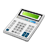Other TopicsCalculus and Higher MathBasic Calculator UseScientific Calculator UseComputer UseComputer ProgrammingGroup Problem SolvingMental MathInductive/Deductive ReasoningMath CommunicationsMathematical Modeling